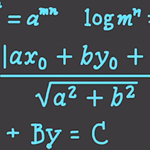# Precalculus 7 : Quadratic EquationsOver these next few Precalculus videos you will be 100% prepared to learn Calculus.

In this video I’ll cover : Quadratic Equations, Quadratic Formula, Completing the Square, Rational Equations, Zero Product Property, Perfect Squares, Converting to Proportions and more.